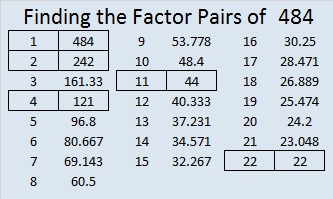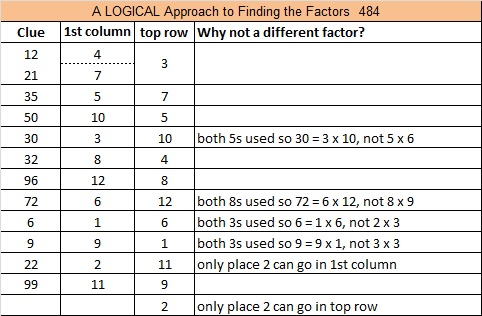# 484 and Level 4

484 is the sum of 14 consecutive primes, and the first of those primes is one of its factors!

11 + 13 + 17 + 19 + 23 + 29 + 31 + 37 + 41 + 43 + 47 + 53 + 59 + 61 = 484Print the puzzles or type the solution on this excel file: 12 Factors 2015-05-04

—————————————————————————————————

• 484 is a composite number.
• Prime factorization: 484 = 2 x 2 x 11 x 11, which can be written 484 = (2^2) x (11^2)
• The exponents in the prime factorization are 2 and 2. Adding one to each and multiplying we get (2 + 1)(2 + 1) = 3 x 3 = 9. Therefore 484 has exactly 9 factors.
• Factors of 484: 1, 2, 4, 11, 22, 44, 121, 242, 484
• Factor pairs: 484 = 1 x 484, 2 x 242, 4 x 121, 11 x 44, or 22 x 22
• 484 is a perfect square. √484 = 22—————————————————————————————————This site uses Akismet to reduce spam. Learn how your comment data is processed.